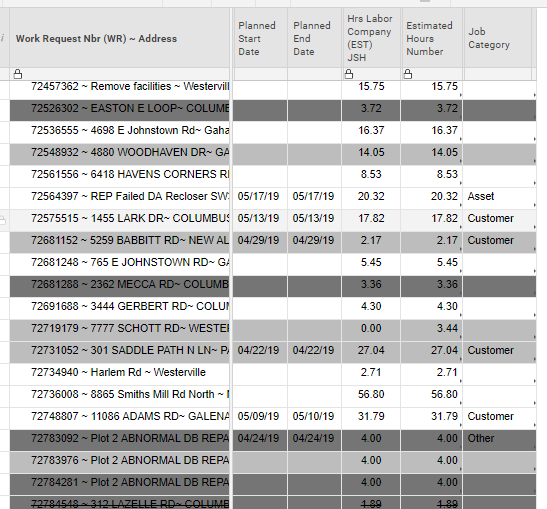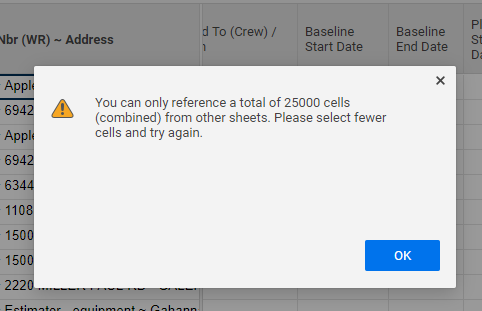# SUMIFS of a value that falls between a rolling date range

Good Morning,

I am struggling with the SUMIFS function. I have a sheet that contains job orders. The job orders have a planned start date. The jobs are also categorized by Job Type. I have the following columns:

Job Type

Planned Start

JSH *job site hours*

What I need is to calculate the sum of total JSH if the job falls within a specific job type AND is scheduled from TODAY and three weeks out. This data set will change and feed a dashboard to see within a rolling 3 week period how many hours are being scheduled within that time frame.

=SUMIFS([Estimated Hours Number]:[Estimated Hours Number], [Job Category], "Customer", AND([Planned Start Date] = Today),([Planned Start Date] <=3 weeks from today)) <= not sure how to set that date calculation

Thank you!

«1

• Three weeks from today is 21 days from now.  Or, TODAY()+21.

• Give this a go...

=SUMIFS([Estimated Hours Number]:[Estimated Hours Number], [Job Category], @cell = "Customer", [Planned Start Date]:[Planned Start Date], AND(@cell >= TODAY(), @cell<=TODAY(21))

• Good Morning - I tried the following. This is my first time using the @cell function so please bare with me. The following gave me "unparseable":

=SUMIFS([Estimated Hours Number]:[Estimated Hours Number], [Job Category], "Customer", AND([Planned Start Date], = TODAY(),([Planned Start Date], <= TODAY(21)))

=SUMIFS([Job Category]:[Job Category], @cell ="Customer", ([Planned Start Date]:[Planned Start Date], AND@cell >= TODAY(), @cell <=TODAY(21)), [Estimated Hours Number]:[Estimated Hours Number])

1. When writing the logic - does the formula first need to find the conditions then sum the range last or does it matter?

2. I took out the time frame out of the equation to do a basic SUMIF and still isn't resolving:

=SUMIF([Job Category]:[Job Category], @cell="Customer", [Estimated Hours Number]:[Estimated Hours Number])

at this point -if the formula is correct - then is it the data set I am pulling from in the sheet?

Thank you!

• The syntax for a SUMIFS and a SUMIF are different. For a SUMIFS it looks something like this...

=SUMIFS(range_to_sum, criteria_range_1, criteria_1, criteria_range_2, criteria_2, .............................)

One thing I did forget to mention and include a solution for...

When referencing a date column like this, and blanks or texts anywhere within the range will also throw an error. To account for this we can use an IFERROR statement and then use a DATE outside of the range.

I also accidentally missed finishing out a range. That was totally my fault and why my first formula came back as unpareseable. Here is a corrected formula to try...

=SUMIFS([Estimated Hours Number]:[Estimated Hours Number], [Job Category]:[Job Category], @cell = "Customer", [Planned Start Date]:[Planned Start Date], AND(IFERROR(@cell, TODAY(-1)) >= TODAY(), IFERROR(@cell, TODAY(22)) <=TODAY(21)))

• Thanks Paul. The formula still isn't working. If I have blanks in any other column range within that formula, will it create a unparseable error? I will have rows that do not have a category or JSH.

• Can you post the exact formula you are using along with a screenshot of the data you are referencing?

• This formula is in my data set sheet (where I have data for dashboard widgets):

=SUMIFS([Hrs Labor Company (EST) JSH]:[Hrs Labor Company (EST) JSH], [Job Category]:[Job Category], @cell = "Customer", [Planned Start Date]:[Planned Start Date], AND(IFERROR(@cell, TODAY(-1)) >= TODAY(), IFERROR(@cell, TODAY(22)) <=TODAY(21)))

The snapshot includes the columns that I use. There are more columns but they are hidden.

Thanks• And you're getting an #UNPARSEABLE error? Your formula seems to be in order. I don't see any extras tucked in anywhere or anything.

How are the numbers in the JSH column populated?

Are both columns housing dates formatted as date type columns?

How are those dates being populated?

How are the cells in the Category column populated?

• Good Morning Paul. To answer your questions - yes the result keeps coming back unparseable.

1. The numbers in the Estimated Hours Number column populated is an odd column. It contains a pretty lengthy IF formula written by another group. I was advised to use this column. However, the column labeled: Hrs labor Company (EST) JSH is data coming from the uploader from our internal company database. That property is Text/Number and is the column I would rather use. However, I did put that column reference in and the result still came back unparseable.

2. The Planned Start/End Date columns are listed as Date properties.

3. The dates are being populated by manual entry from the enduser.

4. Category column is manual entry (via drop down option).

• Is the formula on the same sheet as the data, or is it on a different sheet?

• The formula I am trying to run is on a different sheet. The sheet I add all my formulas to are used for dashboard purposes. The original master sheet that the uploader is connected to continuously adds new rows. So I don't want to put any total calculations in those sheets.

• Ah. that would be the problem then. You will need to use cross sheet references.

Whenever you get to a range [Column Name]:[Column Name] use the link in the helper box that appears below the formula as you type that says "Reference Another Sheet".

Next you will select the sheet that you are pulling data from and then select the range. In this case we are looking at the entire column, so you can just click on the column header.

At this point I recommend changing the range names to specify the sheet and column name for future troubleshooting.

Once you click on the button that says "Insert Reference" it will take you back to the formula builder and you will see your range now appears as {Sheet Name Range Whatever You Typed In}.

Do this for each of the ranges, and you should be squared away.

• Paul,

That is what I initially started to do until I got the following error message...

I am doing a SUMIFS with first condition job category, second condition date, with a sum return of JSH. Apparently, when I highlighted the second condition, I reached a max cell limit?

However, the first condition = 1320 cell count

Second condition = 1320 cell count

Total = 2640 < 25000

Is there a reason why this error is coming up?

Thanks,

Veronica• Are you making sure to select ONLY the column needed for each range? Are you referencing other sheets in other formulas there?

• Yes, I am only selecting the column only. And one cell with the formula is the only cell reference to it.

## Help Article Resources

Want to practice working with formulas directly in Smartsheet?

Check out the Formula Handbook template!